# Texas Go Math Kindergarten Lesson 2.4 Answer Key Count Forward and Backward to 5

Refer to our Texas Go Math Kindergarten Answer Key Pdf to score good marks in the exams. Test yourself by practicing the problems from Texas Go Math Kindergarten Lesson 2.4 Answer Key Count Forward and Backward to 5.

## Texas Go Math Kindergarten Lesson 2.4 Answer Key Count Forward and Backward to 5

Explore

DIRECTIONS: Look at the cube towers. Count how many cubes are in each tower. Write the number.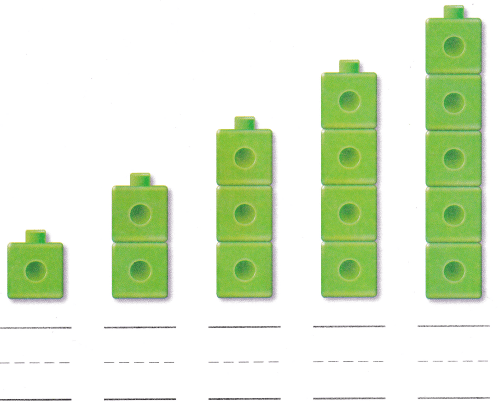Explanation:
Counted the cubes and according to the tower
and written the numbers

Share and Show

DIRECTIONS: Look at the books. Place a counter on each book as you count. Write the number. Start with 5 and write the numbers in order as you count backward.

Question 1.Explanation:
Placed a counter on each book as we count.
Written the number.
Started with 5 and written backwards

DIRECTIONS: 2. Look at the lunchboxes. Write the missing numbers in order by counting forward. 3. Look at the backpacks. Write the missing numbers in order by counting backward.

Question 2.Explanation:
Written the missing numbers
traced the numbers from 3 to 5

Question 3.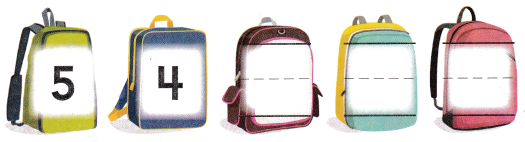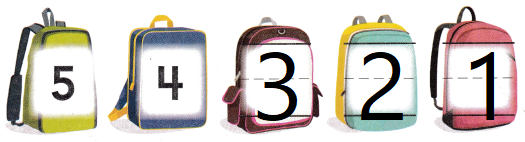Explanation:
Written the missing numbers
traced the numbers from 3 to 1

HOME ACTIVITY • Hove your child count forward to five, putting up one finger at a time. Then hove him or her count backward from 5, putting down one finger at a time.Explanation:
Written the  numbers to count
traced the numbers from 1 to 5

DIRECTIONS: 4. Ted has 5 movie tickets. He wants to put the tickets in order. Draw the movie tickets. Label each ticket with a number so that Ted will be counting forward. 5. Choose the correct answer. Count the cubes in each cube train. Which order do they show?

Problem Solving

Question 4.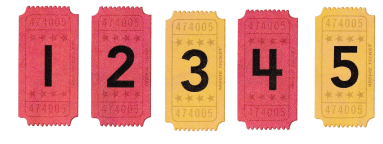Explanation:
placed the cards in order
and written then from 1 to 5

Question 5.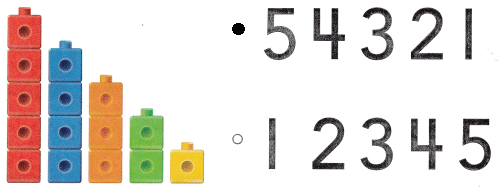Explanation:
Bubbled the correct pattern

### Texas Go Math Kindergarten Lesson 2.4 Homework and Practice Answer Key

DIRECTIONS: 1. Look at the banners. Write the missing numbers in order by counting forward. 2. Look at the books. Write the missing numbers in order by counting backward. 3. Place crayons as shown. Write the numbers as you count backward.

Question 1.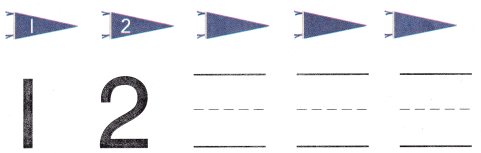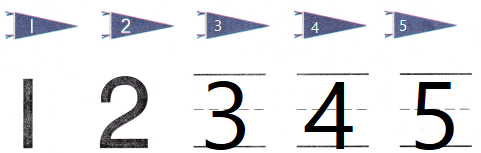Explanation:
Written the missing numbers in order by counting forward.

Question 2.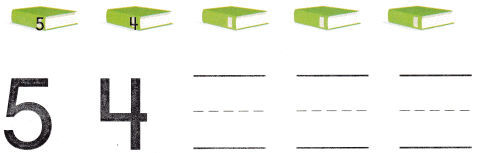Explanation:
Written the missing numbers in order by counting backward.

Question 3.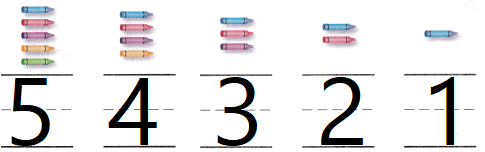Explanation:
Placed crayons as shown.
Written the numbers we  count backward.

DIRECTIONS: Choose the correct answer. 4. Count the cubes in each cube train. What order do they show? 5. Count the counters in each column. What order do they show?

Question 4.Explanation:
Written the numbers in order
Cubes are placed in forward counting

Question 5.